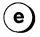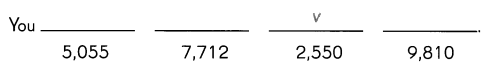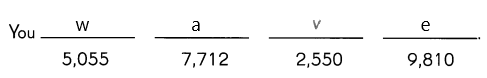# Math in Focus Grade 4 Chapter 3 Practice 1 Answer Key Multiplying by a 1-Digit Number

Go through the Math in Focus Grade 4 Workbook Answer Key Chapter 3 Practice 1 Multiplying by a 1-Digit Number to finish your assignments.

## Math in Focus Grade 4 Chapter 3 Practice 1 Answer Key Multiplying by a 1-Digit Number

Multiply 962 by 6 and find the missing numbers.

Example
Step 1
2 ones × 6 = 12
= 1 ten 2 ones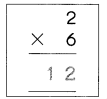Question 1
Step 2
6 tens × 6 = ___ tens
= ___ hundreds ___ tens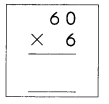6 tens × 6 = 36 tens
= 3 hundreds 6 tens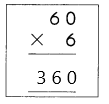Question 2.
Step 3
9 hundreds × 6 = ___ hundreds
= ___ thousands ___ hundreds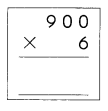9 hundreds × 6 = 54 hundreds
= 5 thousands 4 hundreds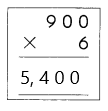Question 3.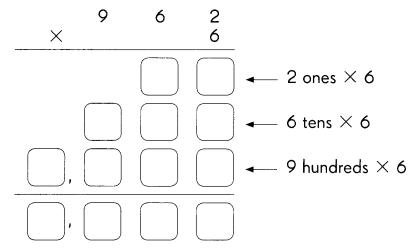962 × 6 = 5,772.

Explanation:
The multiplication of 962 × 6 is 5,772.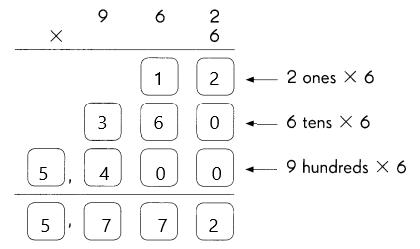Multiply 9,086 by 7 and find the missing numbers.

Question 4.
Step 1
6 ones × 7 = _____ ones
= _____ tens _____ ones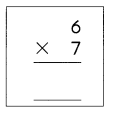6 ones × 7 = 42 ones
= 4 tens 2 ones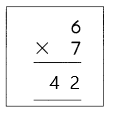Question 5.
Step 2
_____ tens × 7 _____ tens
= ______ hundreds ______ tens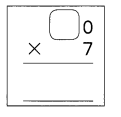8 tens × 7 =  56 tens
= 5 hundreds 6 tens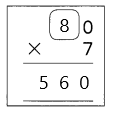Question 6.
Step 3
_____ hundreds × 7 = _____ hundreds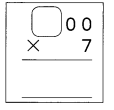0 hundreds × 7 = 0 hundreds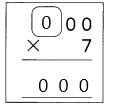Question 7.
Step 4 _____ thousands × 7 _____ thousands
= ______ ten thousands ______ thousands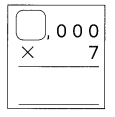9 thousands × 7 =  63 thousands
= 6 ten thousands 3 thousands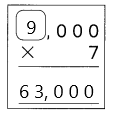Question 8.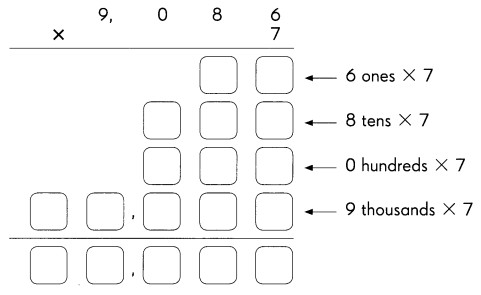9,086 × 7 = 63,602.

Explanation:
The multiplication of 9,086 × 7 is 63,602.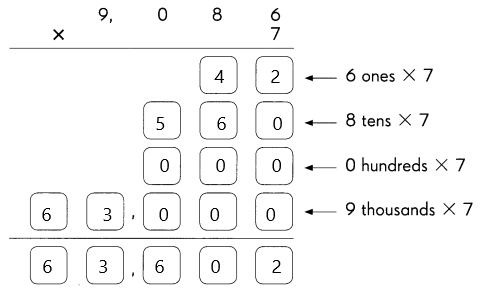Multiply.

Example.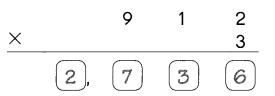Question 9.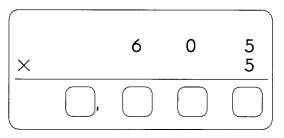605 × 5 = 3,025.

Explanation:
The multiplication of 605 × 5 = 3,025.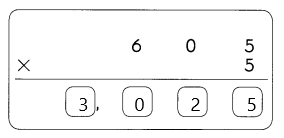Question 10.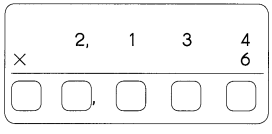2,134 × 6 = 12,804.

Explanation:
The multiplication of 2,134 × 6 = 12,804.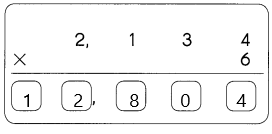Question 11.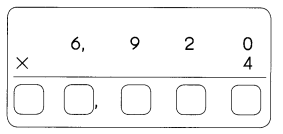6,920 × 4 = 27,680.

Explanation:
The multiplication of 6,920 × 4 = 27,680.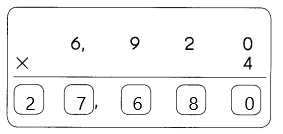Question 12.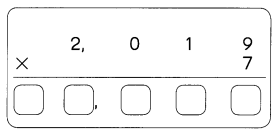2,019 × 7 = 14,133.

Explanation:
The multiplication of 2,019 × 7 = 14,133.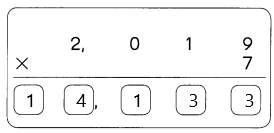Question 13.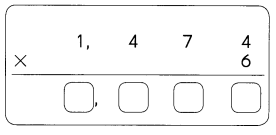1,474 × 6 = 8,844.

Explanation:
The multiplication of 1,474 × 6 is 8,844.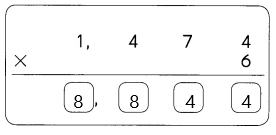Question 14.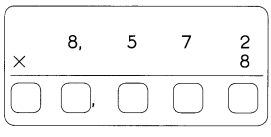8,572 × 8 = 68,576.

Explanation:
The multiplication of 8,572 × 8 is 68,576.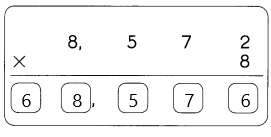Question 15.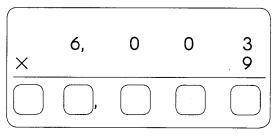6,003 × 9 = 54,027.

Explanation:
The multiplication of 6,003 × 9 is 54,027.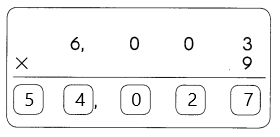Find each product. Then solve the riddle.

Example
425 × 2 = 2,550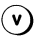Question 16.
964 × 8 = ____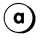964 × 8 = 7,712.

Explanation:
The multiplication of 964 × 8 is 7,712.

Question 17.
682 × 5 = ____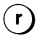682 × 5 = 3,410.

Explanation:
The multiplication of 682 × 5 is 3,410.

Question 18.
1,685 × 3 = ___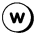1,685 × 3 = 5,055.

Explanation:
The multiplication of 1,685 × 3 is 5,055.

Question 19.
1,936 × 4 = ___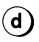1,936 × 4 = 7,774.

Explanation:
The multiplication of 1,936 × 4 is 7,774.

Question 20.
3,270 × 3 = ___